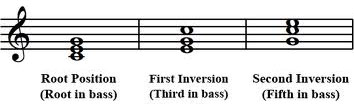A# inversionC major inversions.

An inversion of a chord is the removal of the root from the bass to an upper part (an octave higher).

Consider the C major triad C-E-G. In a first inversion the third becomes the bass (E-G-C or E-C-G). In a second inversion the fifth becomes the bass (G-E-C or G-C-E). Because the three notes have the same key-center and tonality, they have the same name (C major, in this case) but a different sound and sense of motion.

A third inversion is when in a four-note chord the fourth note becomes the bass. (For example, in the G-B-D-F, third inversions would be F-G-B-D and F-B-G-D.)

The inversion of an interval involves moving the lower note to an octave higher. For example, the inversion of the perfect fifth C-G results in the perfect fourth G-C. The rules for inverting intervals are:

• Perfect intervals always invert to other perfect intervals.
• Fourths and fifths invert to each other.
• Major intervals and minor intervals invert to each other.
• Thirds and sevenths always invert to each other.
• Diminished and augmented intervals invert to each other, e.g. C-F♯ (augmented 4th) inverts to F♯-C (diminished 5th)